## How to Use SWITCH function in Google Sheets? (With Examples)

Switch Function in Google Sheets: When an expression is tested against a list of cases, the Switch function returns the value of the first matching case, with an optional default value if no other conditions are matched. The Switch function also comes in handy, since it is very easy to read and understand even if we use multiple conditions. Let us understand how to use the Switch function with the help of Google Sheet tips provided on this page.

## SWITCH Function Syntax in Google Sheets

The syntax for using SWITCH function in Google Sheets are given below:

=SWITCH(expression, case1, value1, [case2, value2, …], [default])

• Expression: This specifies the values that the function will examine. This can be a specific cell reference, such as “B2, C2, A2 and so on.”
• Cases: The function looks for an exact match by comparing the value of a case to the expression.
• Values: This is what the function returns in the cell if the expression and the corresponding case are exact matches. The text “No” provides a good example of a return value.
• Default: This is optional. If none of the cases match the expression, an optional value will be returned as the last parameter.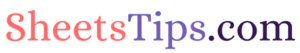### Using SWITCH Function with Two Conditions

Let us create the SWITCH function using the topmost row as a starting point. We are looking at row 2 in this example dataset.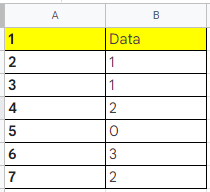Make a list of the case/value pairs. The first case/value pair in this example is 0 and “No,” whereas the second case/value pair is 1 and “Yes.”

So, our SWITCH function formula will be =SWITCH(B2,0,”No”,1,”Yes”)

Now consider the following dataset and follow the steps listed below to use the SWITCH function:

• Step 1: Move to cell C2.
• Step 2: Now use the formula =SWITCH(B2,0,”No”,1,”Yes”).
• Step 3: Press the “Enter” button.
• Step 4: Now drag the fill handle to other cells and you will see the results.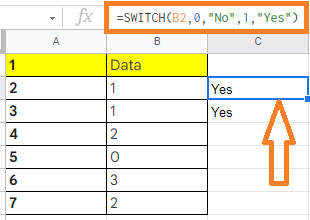### Using SWITCH Function with One Condition and 1 Default in Google Sheets

A SWITCH function will return an error if you don’t account for all possible case matches.

Fortunately, you can add the optional “default” value (also known as a fall-back value) to the end of the statement to return a value if no matching case is found.

If we’re using the SWITCH function to determine a student’s pass/fail status based on grades, we may set it to return “Fail” when an “F” case is detected, and “Pass” in all other cases.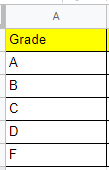So, our SWITCH function, in this case, will be =SWITCH(A2,”F”,”Fail”,”Pass”)

Now follow the steps as listed below to use the SWITCH Statement with default value:

• Step 1: Move to the row where you would like to use the SWITCH function. In this example, we are using the 2nd row, cell C2.
• Step 2: Now enter the formula as =SWITCH(A2,”F”,”Fail”,”Pass”).
• Step 3: Press the “Enter” button. You will see the results.
• Step 4: Now drag the formula applied cell to other rows and you will see the results.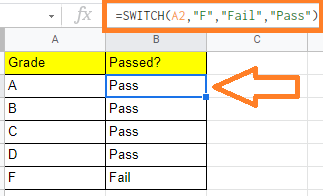### Using SWITCH Function with Multiple Condition and 1 Default in Google Sheets

Let us look at how to use the SWITCH function with several case/value pairs and a default option one more time. For that, we will use the same example dataset which we have used previously.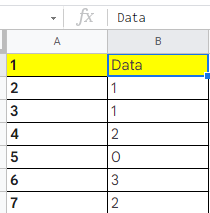For the above-pictured dataset, follow the steps listed below to use Switch Function:

• Step 1: Start building the SWITCH function in Google Sheets. For that move to cell A2. So our SWITCH function conditions are:
• If data contains “0”, then it should return “No“.
• If data contains “1”, then it should return “Yes“.
• If it does not contain both 0 and 1, then it should return Other.
• Step 2: So our SWITCH function formula will be =SWITCH(B2,0,”No”,1,”Yes”,”Other”). Enter the same formula in Cell B2.
• Step 3: Press the “Enter” button.
• Step 4: You will see the results. Now drag the formula applied cell to other rows to apply the SWITCH function and see the results.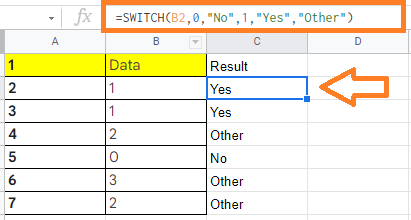## How to Use Wildcards in Google Sheets with Examples?

Every Google Spreadsheet user will have to learn how to use the Wildcard characters. Generally, in Google Sheets, we can combine wildcard characters with functions to perform a variety of useful opeartions. In this article, let us explore how to use wildcards with the help of Google Sheet tips provided on this page.

## What is Wildcard in Google Sheets?

When you use a wildcard symbol, you can represent any character by using that symbol instead of the actual characters themself. For specific results, they are often used in combination with other query functions like conditional functions, search and lookup functions, and filtering.

In Google Sheets, there are three wildcard characters and they are:

• Asterisk (*): Any character or any number of characters is represented by an asterisk (*).
• Question Mark (?): One character is represented by the question mark wildcard (?).
• Tilde (~): You can also use the Tilde (~) to tell Google Sheets not to use any wildcard characters (such as * and ? ). Google Sheets will know if you search for “m~?” and not use the question mark as a wildcard in this case if you use “m?” as an example in your search.

### How to Use Wildcards Using SUMIF Function? (* Wildcard)

Let us consider the following dataset. Here we want to calculate the October salary of HR department employees.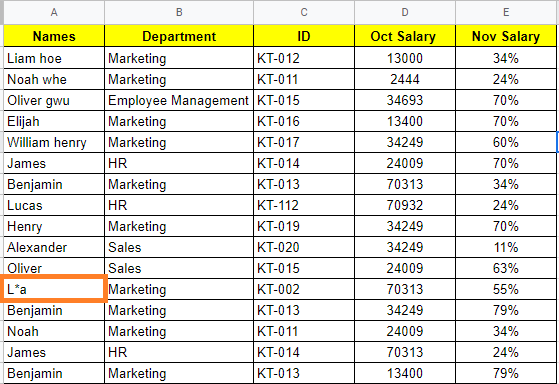Now follow the steps listed below to use Wildcards using SUMIF Function in Google Sheets:

• Step 1: Move to the cell where we want to get the results. In our case, we are using cell G2.
• Step 2: Now type the formula as “=SUMIF(B2:B17, “HR*”,D2:D17)“.
• Step 3: Press the “Enter” button.

Now you will see the results as shown below.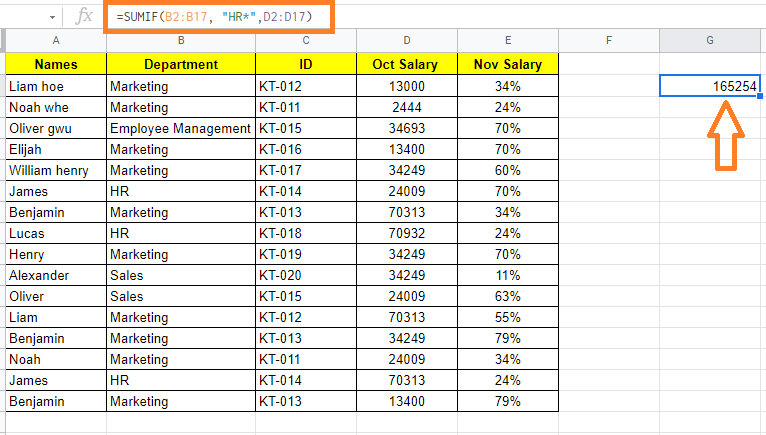Formula Explanation:

In the above example, we have used the condition “HR*” which means find all the cells which contain the word HR. Now the spreadsheet will not search for the exact match, but it searches all the cells which contain the word “HR” along with other characters.

Once similar texts are found, the SUMIF function will calculate the total salary value

When a match is found, the SUMIF function adds the October salary value corresponding to the matching cell to the list of Total Salary values that have been selected. The SUMIF function sums up the selected Total salary values and displays the result in cell G2 once it has finished going through all of the models.

In other words, the wildcard ‘*’ aided in the discovering of various variations of the search term ‘HR’ in column B.

### Using Wildcards to Filter Data Based on Conditions in Google Sheets (? Wildcard)

Wildcards can also be used to quickly and easily filter data based on a condition. Let us start with the same dataset as shown in the image below:

Assume you want to filter the data so that only cells beginning with the letter K and ending with the number 2 are visible. This is very easy to execute by using the question mark wildcard with the help of the steps given below:

• Step 1: Select the range of cells that you would like to filter.
• Step 2: Click on the “Data” menu from the toolbar and choose “Create a Filter” from the drop-down menu.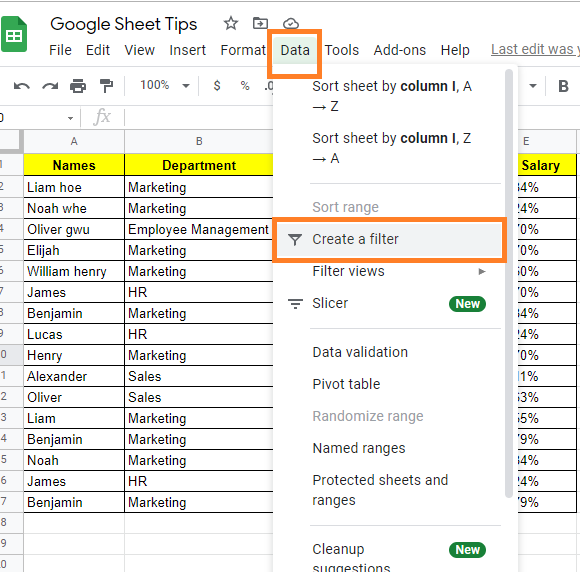• Step 3: Now click on the “Filter” icon against the “ID” column.
• Step 4: A sub-menu will open up on the screen. Select the “Filter by Condition” option.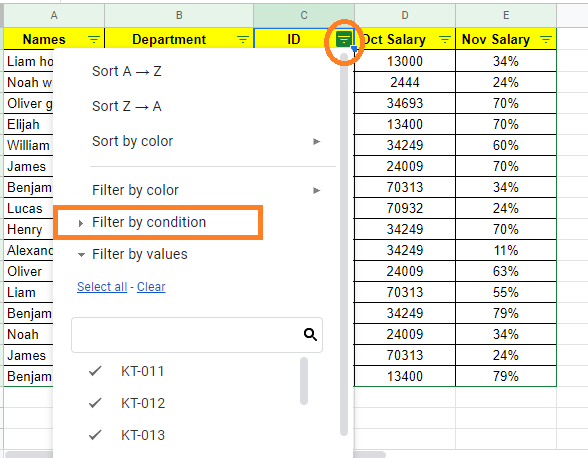• Step 5: Now choose “Text Contains” from the drop-down menu.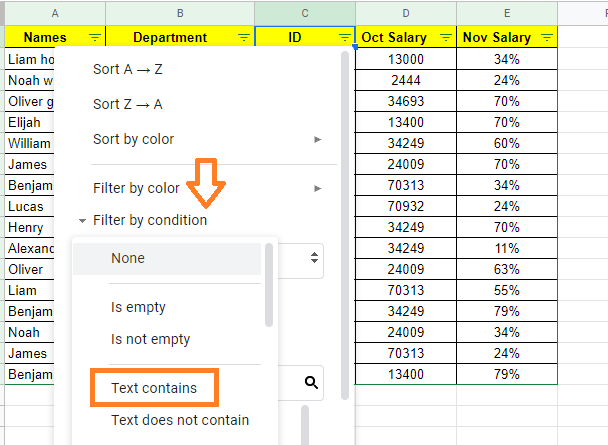• Step 6: Here a new input box will appear on the screen. Here you will have to enter the formula.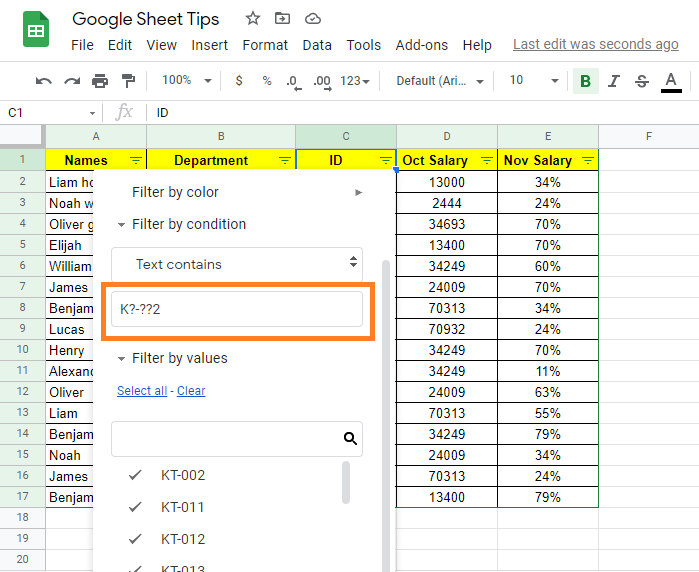• Step 7: Type the search string or formula as ‘K?-??2‘.
• Step 8: Click on the “Ok” button and you will see the results.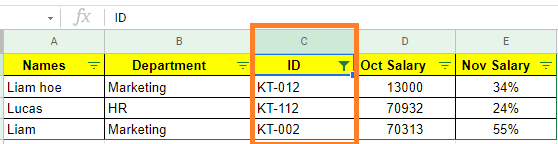### How to Use Wildcards Using COUNTIF Function? (Tilde ~)

Although the tilde wildcard (~) isn’t used very often, it’s still useful to know how to utilize it. Let us understand how to use the Tilde ~ wildcard using the COUNTIF function. Consider the following dataset. Here we need to find out the string which exactly has the word “L*a”.Let us understand how to achieve the same with the help of the steps given below:

• Step 1: Move to the cell where you would like to extract the string which has the word “L*a”.
• Step 2: Now enter the formula using the COUNTIF function as =COUNTIF(A2:A17,“L~*a”)
• Step 3: Press the “Enter” button. Now you will see the results as shown below.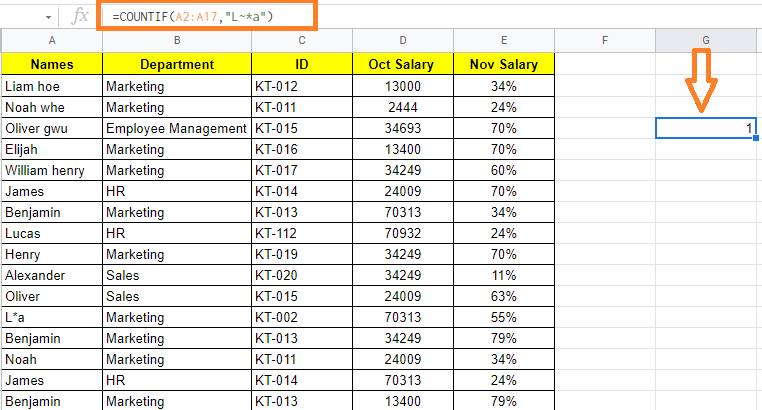## How to Use Filter Functions in Google Sheets with Examples

Google Sheets comes with various functions such as VLOOKUP, IMPORTRANGE, REGXREPALCE, and so on. The FILTER function is one such amazingly strong one where we can perform various options without using the FILTER Views.

## Syntax for Using Filter Functions in Google Sheets

The syntax for Filter Functions in Google Sheets are given below:

FILTER(rangecondition1[condition2, …])

• range – the filtered data should be within this range.
• condition 1: The condition on which the data is filtered is condition 1.
• condition2: additional conditions that the data is filtered based on.

It’s important to remember that the FILTER function only returns data that meets all of the specified criteria.

### Highlights of Filter Functions in Google Sheets

• The FILTER function returns an array as a result. This means you won’t be able to remove a portion of the result.
• Filter Functions will produce a #N/A error if there is no match based on the conditions you gave.
• The formula will return a REF error if any cell that is expected to be filled by the result of the FILTER function is already filled.

Now that we are aware of the syntax of FILTER functions in Google Sheets. In the below section, let us understand how to use Filter Functions with examples.

### Filter Functions using Single Condition in Google Sheets

Let us consider we have an employee dataset and we need to filter only the employee who are belonging to the marketing department. Now follow the steps listed below to achieve the same:

• Step 1: Move to the cell where you would like to use the Filter Functions.
• Step 2: Now enter the formula using Filter function syntax. In our case, we are using the formula as “=FILTER(\$A\$2:\$C\$17,\$B\$2:\$B\$17=”Marketing”)“.
• Step 3: Press the Enter button. Now you will see the data being filtered out without using inbuilt filter options.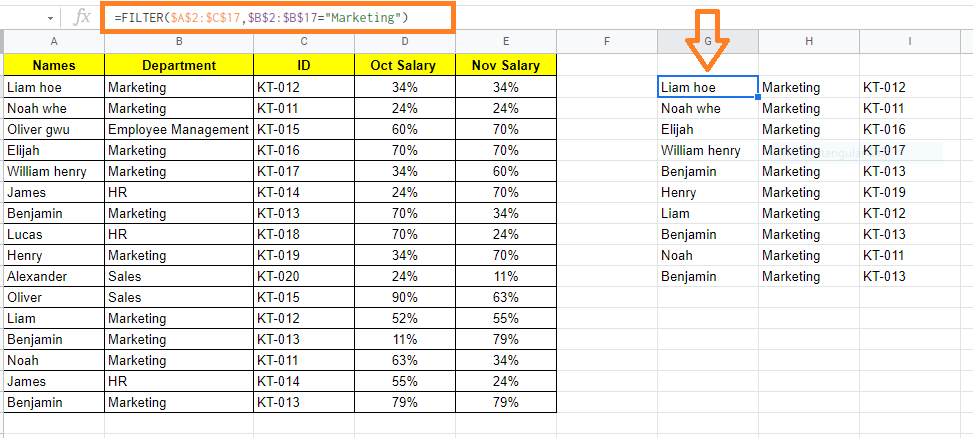### Filter Functions using Multiple Condition in Google Sheets

Let us say, in the same dataset, I want to extract the employee data who are working under the marketing department and along with it, I also want to extract whose salary was greater than 50%.

Now follow the steps listed below to achieve the same.

• Step 1: Move the cell where you want to extract the data using the filter function.
• Step 2: Now use the formula as “=FILTER(\$A\$2:\$C\$17,\$B\$2:\$B\$17=”Marketing”, \$D\$2:\$D\$D17>50%)
• Step 3: Press the “Enter” button. The results will be as shown in the image below.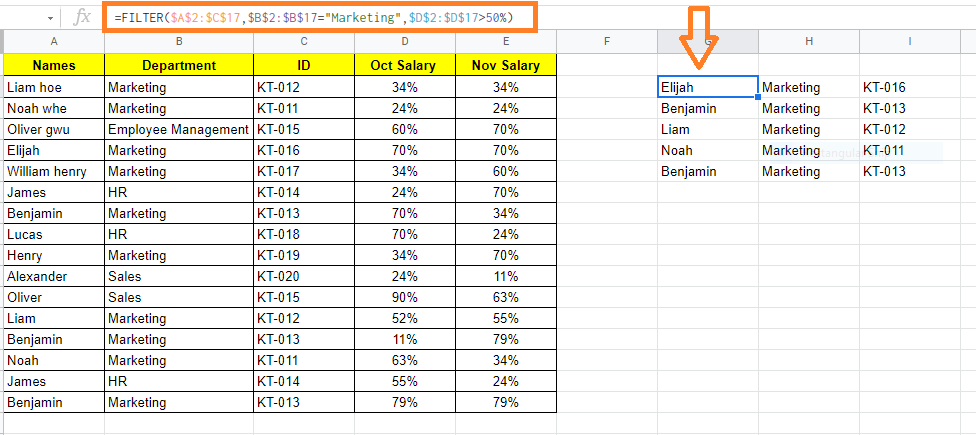### Filter Functions using Odd/Even Condition in Google Sheets

It’s really simple to extract all the even-numbered rows or the odd-numbered rows from a dataset using the FILTER function.

Let us consider the same dataset where we need to filter even rows and odd rows using Filter functions. To get this done, follow the steps listed below.

• Step 1: Move to the cell where you would like to use the Filter Function.
• Step 2: Now use the formula “=FILTER(\$A\$2:\$D\$17, MOD(Row(A2:A17)-1,2)=0)”.
• Step 3: Press the “Enter” button. This will filter all the even-numbered rows as shown below.

Since we have represented row 1 as headers, Google Sheets ignores row 1 and starts counting from Row 2.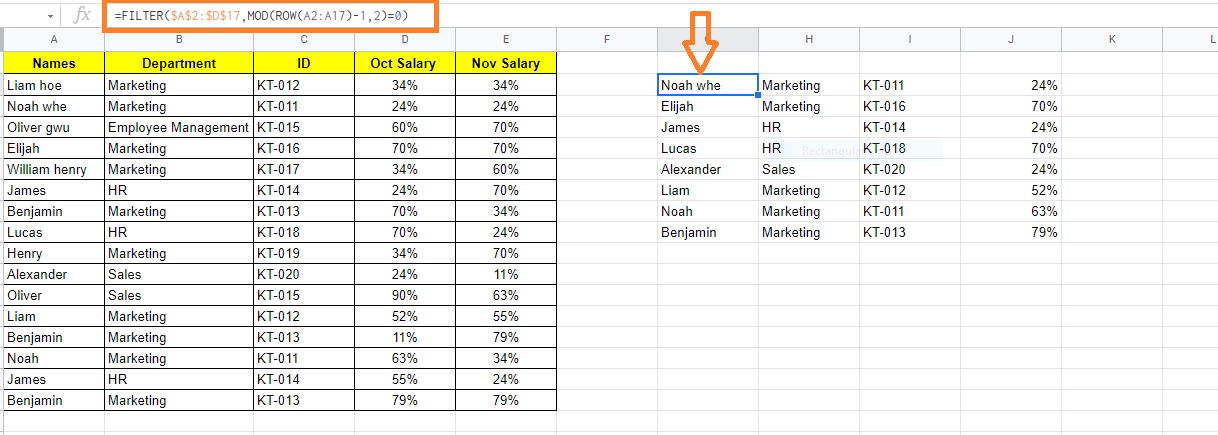To filter the odd number of rows, use the formula =FILTER(\$A\$2:\$D\$17, MOD(Row(A2:A17)-1,2)=1)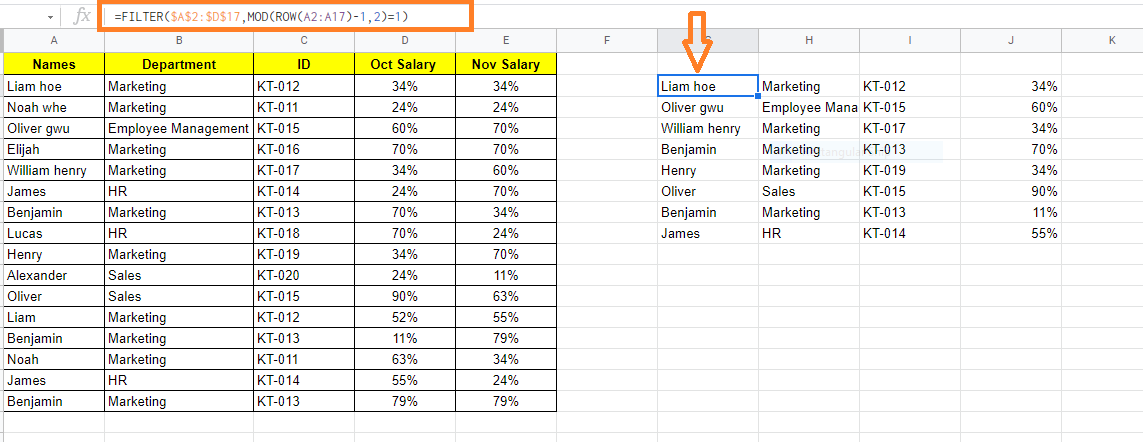### Filter Functions using ABOVE/BELOW Average in Google Sheets

You can apply conditions like the data points (or records) should be above or below average when using the FILTER function.

Let us understand how to use above or below Average functions in Google Sheets by following the steps listed below:

• Step 1: Move to the cell where you would like to use the AVERAGE Function in Google Sheets.
• Step 2: Now enter the formula as “=FILTER(\$A\$2:\$D\$17,\$D\$2:\$D\$17>AVERAGE(\$C\$2:\$D\$17)“.
• Step 3: Press the “Enter” button. You will see the results as shown below.

Here we have used the above Average function.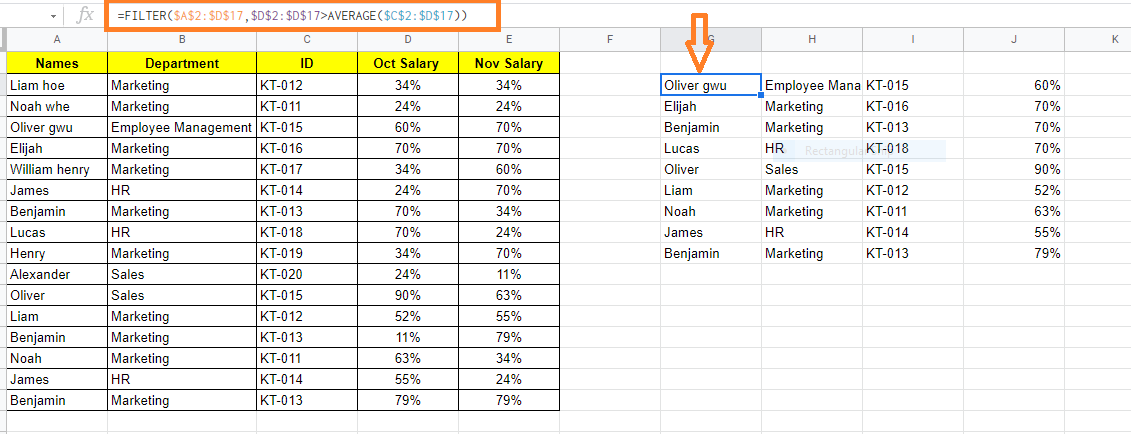The formula to use the below-average function in Google Sheets is =FILTER(\$A\$2:\$D\$17,\$D\$2:\$D\$17<AVERAGE(\$C\$2:\$D\$17).

## How to Convert Time to Decimal in Google Sheets? (Hour, Minute, Seconds in Decimal)

When we are working with Google Sheets, we might fall under certain situations where we need to convert time to decimal values.

For instance, you could want to convert the difference between a task’s start and finish times to a decimal number. This can be used to calculate the number of hours worked. It’s sometimes more obvious to state 4.25 hours worked rather than 4:15 hours worked. It also comes in handy if you need to use the difference value in other calculations later. In this article, let’s understand how to convert time to decimal values with the help of Google Sheet tips provided on this page. Read on to find more.

## Methods to Convert Time To Decimal Values in Google Sheets

There are multiple methods with the help of which we can convert the time to decimal values and they are:

1. Using Time Functions in Google Sheets such as Hour, Minute, Seconds
2. Using TIMEVALUE functions with simple multiplication

### Type 1: Using Time Functions Such as Hour, Minute, Seconds in Google Sheets

HOUR, MINUTE, and SECOND are three-time functions available in Google Sheets. What each of these functions performs is as described in the following sections:

### Hour Function in Google Sheets

This function takes a time value and returns its hour component. If you provide this function the time value “04:15:30,” it will return 4, because there are four hours in the day. The Hour function’s syntax is as follows:

=Hour(time)

Time is either a time value or a reference to a cell that has a time value in this case. You may also use this function to pass the numeric representation of the time and get the proper number of hours.

### Minute Function in Google Sheets

The minute component of a time value is returned by this method. If you provide this function the time value “04:15:30,” it will return 15, because there are 15 minutes in the time. The function’s syntax is as follows:

=Minute(time)

Time is either a time value or a reference to a cell that has a time value in this case. You can also pass the time as a numeric representation.

### Second Function In Google Sheets

This function accepts a time value and returns its second component. For example, if you pass the time value “04:15:30” to this function, it will return 30, because the time is 30 seconds long. The function’s syntax is as follows:

=Second(time)

Time is either a time value or a reference to a cell containing a time value in this context. You can also pass the time as a numeric representation.

### Converting Time to Number of Hours Decimal Format in Google Sheets

Once you know how many hours, minutes, and seconds are in a given time value, you can easily convert it to its decimal equivalent (in hours) by using the following formula:

=HOUR(A2)+MINUTE(A2)/60+SECOND(A2)/3600

We will assume that the time value you would like to convert is in cell A2. When this formula is applied to the time value in cell A2 shown below, the result is 4.258333333.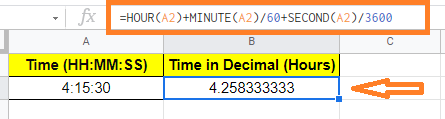We want to convert the number of minutes (15 in our case) to the number of hours using the formula above. Because 60 minutes equals one hour, 15 minutes equals 15/60th of an hour. In other words, it will take 14 minutes or 0.25 hours.

Likewise, 3600 seconds equals one hour. So 30 seconds equals 30/3600th of an hour. In other words, it will take approximately 0.0083333 hours to complete.

We can calculate the total number of hours in the time value by adding all three values. So:

2 hours + 0.25 hours + 0.008333 hours = 4.258333333

### Converting Time to Number of Minutes in Google Sheets

You can use the following formula to convert a time value to its decimal equivalent (in minutes):

=HOUR(A2)*60 + MINUTE(A2) + SECOND(A2)/60

We will assume that the time value you would like to convert is in cell A2. As a result of applying this formula to the time value in cell A2 shown below, the result is 255.5.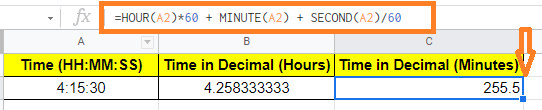### Converting Time to Number of Seconds in Google Sheets

You can use the following formula to convert a time value to its decimal equivalent (in seconds):

=HOUR(A2) * 3600 + MINUTE(A2) * 60 + SECOND(A2)

We will assume the time value you’re trying to convert is in cell A2. As a result, when this formula is applied to the time value in cell A2 below, the result is 15330.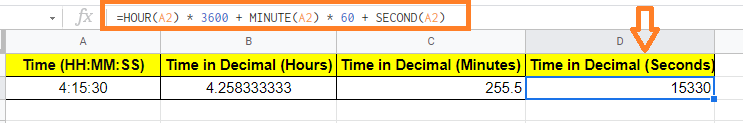### Method 2: Using TIMEVALUE & Multiplication to Convert Time to Decimal in Google Sheets

An alternative process includes multiplying the time value by the number of hours, minutes, and/or seconds in a day. Internally, Google Sheets records time data as decimal numbers, with the value 1.0 representing 24 hours. As a result, we use the value 1/24 to represent one hour. To use this approach to convert a time value to a decimal, we must first obtain its internal value using the TIMEVALUE function.

### What is TIMEVALUE Function?

The syntax for the TIMEVALUE function is:

=TIMEVALUE(time_string)

Here the time_string is a string that contains the time value or a reference to a cell that contains a time value in this case.

This function accepts a time value and returns a fraction of a 24-hour day as a result. If you provide this function the time value “12:00:00,” it will return 0.5 because 12 hours is half a day.

Similarly, if you give this function the time value “06:16:00,” it will return 0.2611111111, which is close to 0.25, because 6 hours 16 minutes is nearly a quarter of a day.

### Converting Time to Number of Hours in Google Sheets

The TIMEVALUE function performs a numerical conversion based on the time value passed to it. Once you have this number, you can simply multiply it by 24 to get the total number of hours in a day. The number of hours in a specific period of time will be displayed in decimal form.

Because there are 24 hours in a day, the TIMEVALUE function’s results must be multiplied by 24 to account for this. Here’s the formula for converting the time value to its decimal equivalent (number of hours):

= TIMEVALUE(A3) * 24

To begin, let’s assume your time value is in cell A3. As a result, entering the time value in cell A3 gives us 4.258333333.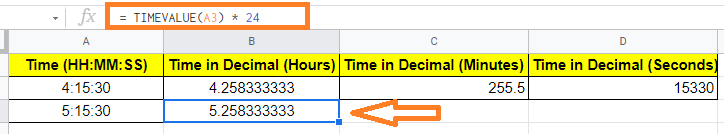### Converting Time to Number of Minutes in Google Sheets

The TIMEVALUE function performs a numerical conversion based on the time value passed to it.

You can then take this number and multiply it by the number of hours in a day. This will show you how many minutes are in the specified time value in decimal form.

Because there are 1440 minutes in a day, we must multiply the TIMEVALUE function’s returns by 1440. How many minutes are in one hour? Here is the formula to calculate how many seconds are in one hour:

= TIMEVALUE(A3) * 1440

To begin, let’s assume your time value is in cell A3. This formula, when applied to the time value in cell A3, gives a value of 255.5.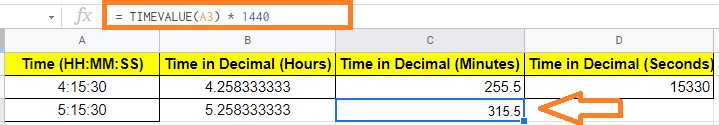### Converting Time to Number of Seconds in Google Sheets

The TIMEVALUE function performs a numerical conversion based on the time value passed to it. Then, all you have to do is multiply this number by the number of seconds in a day. This will show you how many seconds are in the given time value in decimal form.

Because there are 86400 seconds in a day, we need to multiply the TIMEVALUE function’s values by 86400. The following formula can be used to convert the time value to a decimal number (in seconds):

= TIMEVALUE(A3) * 86400

We will assume the time value you’re trying to convert is in cell A3. That is 15330 if you use this formula on the time value in cell A3.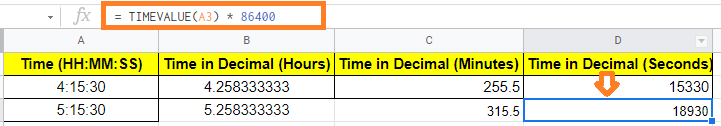## What If Analysis in Google Sheets Using Goal Seek (With Examples)

Google Sheets goal seek feature is a strong data analysis tool. It is essentially an algorithm that allows you to supply the desired output and then returns the value of a given input variable that will assist in achieving the desired output. In this article, let us learn everything about Goal Seek analysis with the help of Google Sheet tips provided on this page.

## Goal Seek Algorithm in Google Sheets

The Goal Seek algorithm simply does a series of what-if calculations, gradually changing the input values until you get the desired result. For example, let’s consider we have employee salary data. If you want to reduce the salary by some percentage, then you can alternate the same by using the Goal Seek tool.

That is you can specify the percentage of salary you to need reduce by mentioning the cells where you need to make the alterations.

A Goal Seek algorithm is made up of three parts:

• A variable whose value is unknown
• A predetermined output value
• To get to the known output, use a formula that includes the unknown input

### How to Use Goal Seek in Google Sheets?

In order to use Goal Seek in Google Sheet, we need to install the Goal Seek add-on function to Google Sheets. The step to install Goal Seek Add-on are given below: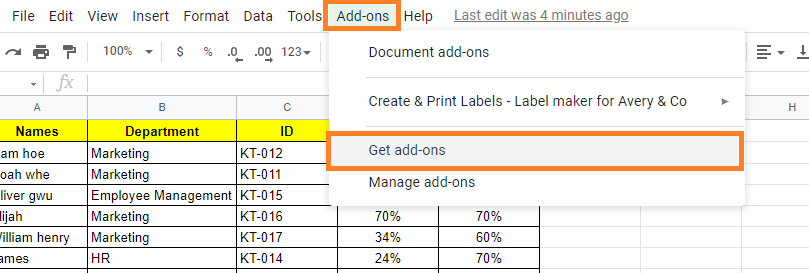• Step 3: In the Google Workspace Masterplace search bar, enter “Goal Seek“.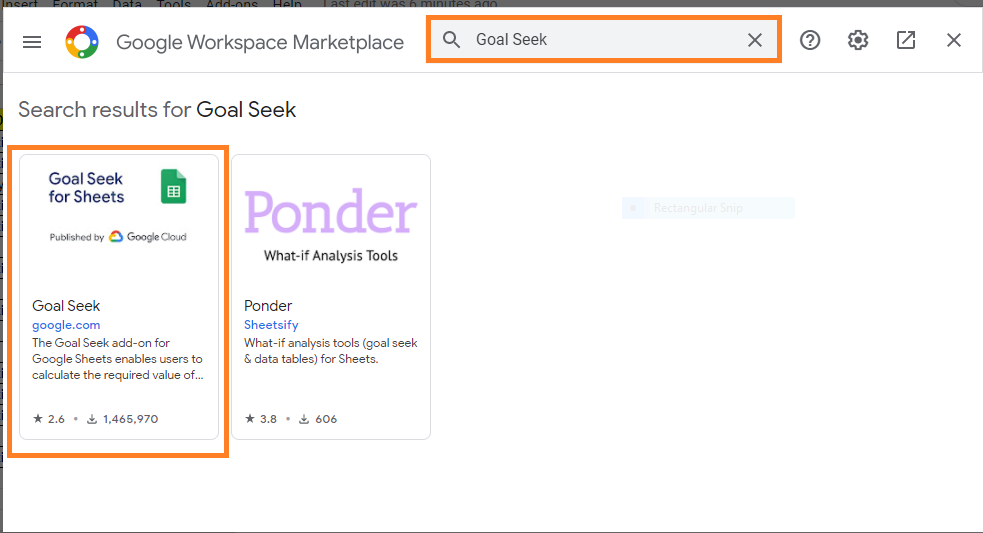• Step 4: The Goal Seek application will pop on the screen. Now click on the app and then hit on the “Install” button.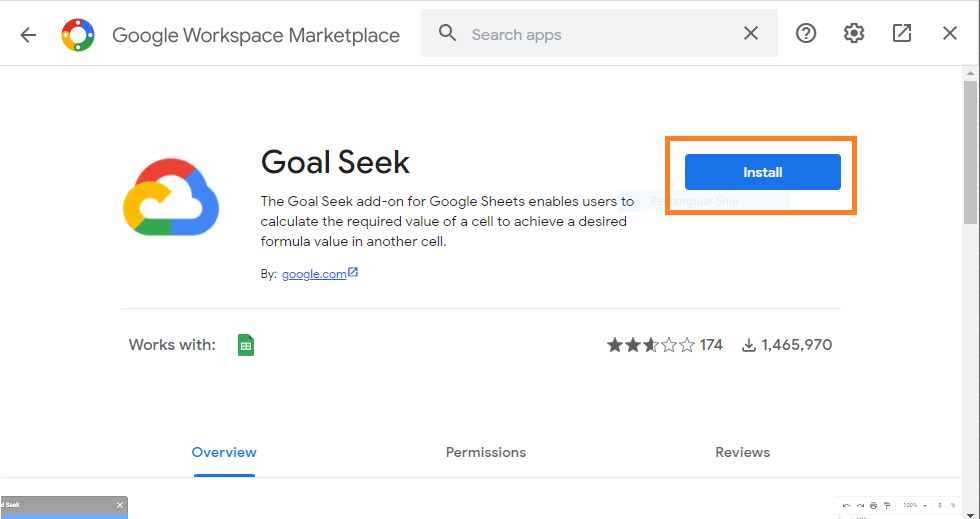• Step 5: To install Goal Seek, you will be prompted for permission. Continue by pressing the Enter key.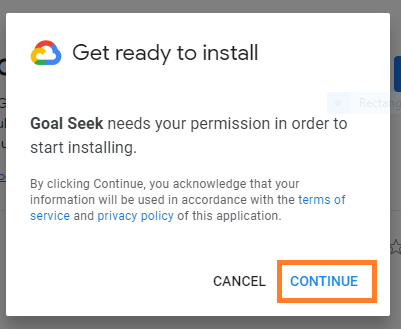• Step 7: The notification ‘Goal Seek has been installed‘ should now appear on the screen. After that, click on the Done button.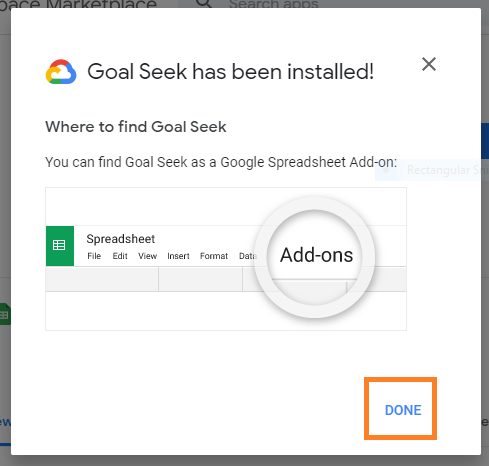• Step 8: The Google Workspace Marketplace window should now be closed. The Goal Seek add-on should now be available as a menu item under the ‘add-ons’ menu.

### How to Enable Goal Seek Window on Google Sheets?

To enable the Goal Seek window in Google Sheets, follow the steps as listed below:

• Step 2: Select “Goal Seek” from the drop-down menu and further select “Open” from the submenu.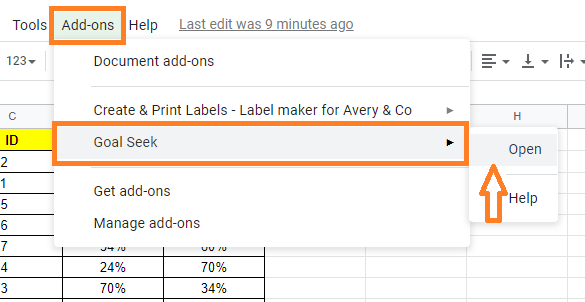• Step 3: You should be able to see the Goal seek window as a sidebar panel of your Google Sheets window once the app has launched.
• Step 4: Now you will see 3 parameters as “Set Cell, To Value, and By Changing Cell“. If you see this, then it means that the Goal Seek window is enabled on Google Sheets.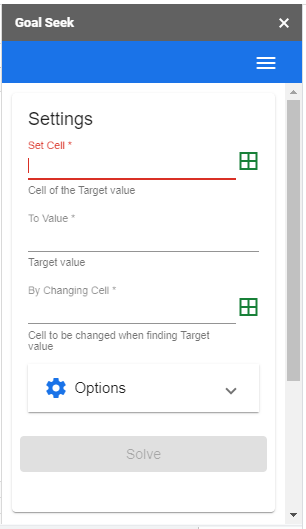### How to Set Up Goal Seek Options?

You may just put in your desired values and let Goal Seek do the rest once the window has opened.

1. Set Cell in Goal Seek

There are two options for providing this information. One method is to type the cell reference of the cell containing the known output value directly into the cell (D17).

Another option is to choose cell D17 and then click the ‘Capture selected cell’ button in the Goal Seek pane’s ‘Set Cell’ field.

2. To Value in Goal Seek

Type your goal value in the box labeled “To Value”. In our case, it is 567832 in our case. Make sure the values you have entered don’t contain currency signs or separators in your text.

3. By Changing Cell

The cell reference for the cell containing the unknown value must be provided here. This reference can be provided in two ways, similar to the Set cell value method. One option is to type the cell reference directly (d4).

Another option is to choose cell d4 and then click the ‘Capture selected cell’ button in the Goal Seek pane’s ‘By changing cell’ field. It’s important that the cell reference entered in the ‘Set cell’ field depends on the reference given in the ‘By changing cell’ field.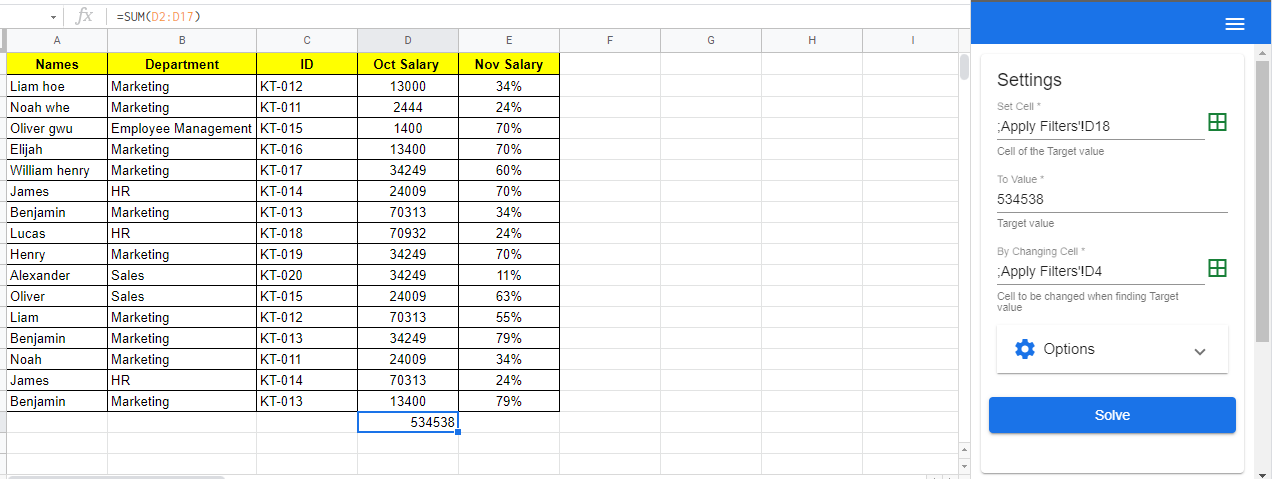### How to Use Goal Seek in Google Sheets?

Once you have decided what you want to put in Goal Seek’s input options, you can move on to the next step:

• Step 1: In the ‘Set cell,’ ‘To value,’ and ‘By altering cell‘ inputs, type the values you need.
• Step 2: Select “Solve” from the drop-down menu.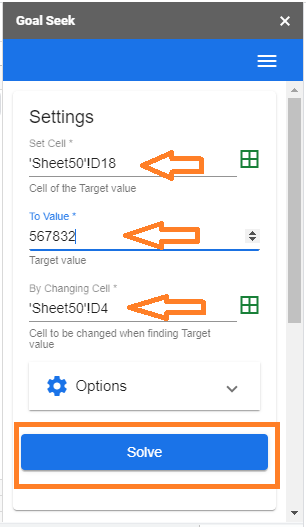• Step 3: Google Sheets will do the necessary calculations and update the values in cells D4 and D17 until the desired value in cell D17 is reached.
• Step 4: You will receive a notification once the Goal Seek is completed, indicating that the required Goal Seek has been accomplished.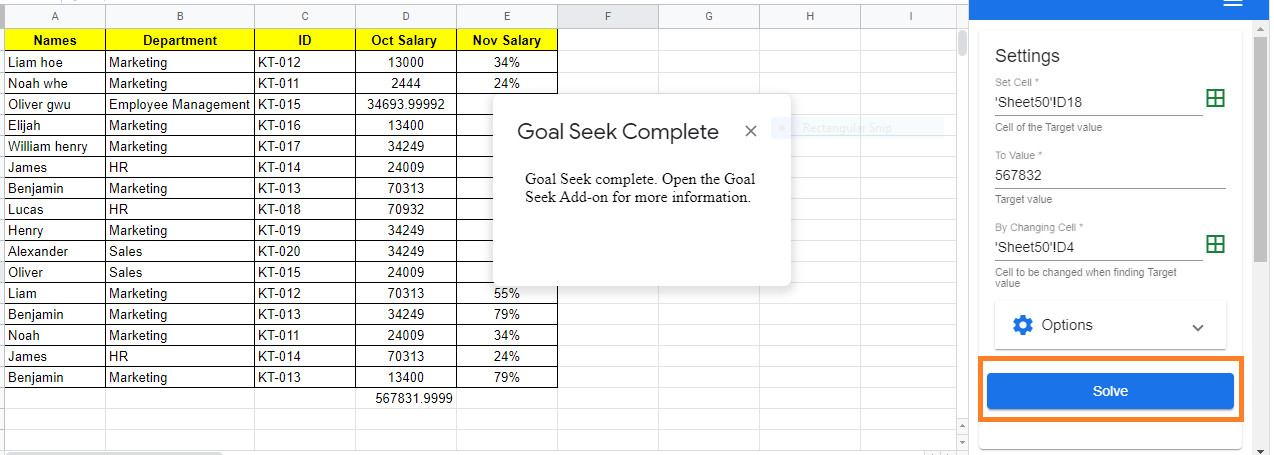You can also scroll down to the bottom of the Goal Seek panel to see the “Solve Status” at this point. Here you will find all of the input values as well as other relevant information such as Goal Seek start time, running time, and so on.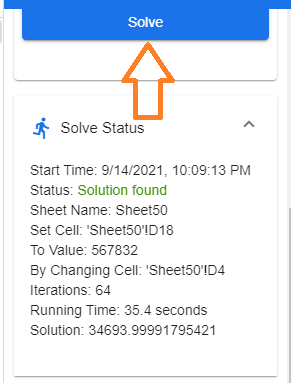## How to Convert Text to Date in Google Sheets? (With Examples)

In Google Sheets, we have n number of options to represents dates. However, in a few instances, the Dates will be understood as text when we are importing files to Google Sheets.  And thus if we want to perform some operations with Dates in Google Sheets, it will lead to improper results or errors. To overcome this issue, we will have to convert text to date. Let’s understand how to convert text to date with the help of Google Sheet tips provided on this page

## How to Find Out If a Date Is Actually a Text Value in Google Sheets?

Using the ISDATE function, you may determine whether a value in a cell is a date or not.

If the value in a cell is a date, then the function returns TRUE otherwise it returns FALSE in Google Sheets.

The valid date will be as follows, we need to specify the day followed by the month and then a year.

Below are other ways to tell if the date is actually a text value,

• If the contents of the cell are aligned to the left, then it is not date but the text value
• If the contents of the cell are aligned to the right, then it is a date.
• In some cases, when you click on the cell, you will see a leading apostrophe to the date in the formula bar, signaling that it is a text value

Example: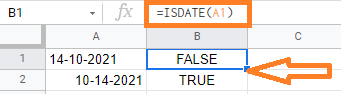In the above example,

• If you notice that the A1 cell is left-handed aligned and also the date format is mentioned in date-month-year, thus the result is FALSE
• If you notice that the A2 cell is right-handed aligned and also date format is mentioned in month-date-year, thus the result is TRUE.

## How to Convert Text to Date in Google sheets?

Once you identify the date is considered as a text in google sheets, then you can convert it to date.

Below are the situations where the date is considered as text,

• A previous user may have worked on the sheet and saved the date values as text, either by appending an apostrophe (‘) to the date value or by changing the date to plaintext format.
• Date formats are understood by the Google sheets based on the region and language settings. When the date format is mentioned in dd-mm-yyyy. Note that Google Sheets accept only date format with mm-dd-yyyy.
• If the user gives an invalid date like, 14102021, then it is considered as a text.

Below are some of the methods where you can convert the text as a date value,

### Using DATEVALUE and TO_DATE Function to Convert Text to Date in Google Sheets

To convert the text format to date in google sheets, you could use any of the DATEVALUE and TO_DATE functions.

The DATEVALUE function takes a date value in any format and returns the same date as a serial number.

Syntax:

DATEVALUE(date_string)

In the above syntax,

• date_string represents the date in TEXT format

The TO_DATE function converts a given serial number to its corresponding date.

Syntax:

TO_DATE(value)

In the above syntax,

• the value represents the number that has to be converted to date format

In order to use the TO_DATE function, we need to call the DATEVALUE function in google sheets.

Example: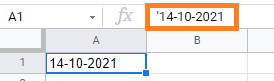In the above example, the date is prefixed with (‘)-apostrophe which means it is considered as TEXT value.

### Using DATE Value Function in Google Sheets

It is important to note that there’s a leading apostrophe, indicating that this is a TEXT value.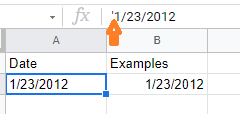To convert this TEXT date to a correct DATE value, use the TO DATE function in combination with the DATEVALUE function, as shown below:

=TO_DATE(DATEVALUE(A2))

The DATEVALUE function will convert the text date to a number value, which will then be converted back to an actual date using the TO DATE function, as shown in the image below: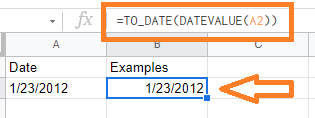### How to Convert Text to Date when Date is in an 8-digit Number Format?

When we import a Google Sheets from a PDF version to a sheet version, the dates will be imported in text format. For example, if your date format is 12/02/2021 in PDF and when you import this version, it will be formatted as 12022021 which is an 8 digit format.

In these cases, we need to convert text to date. Let us understand how to do this with the help of the steps listed below:

• Step 1: Consider the following dataset where we have the Date in 8 digit text format.
• Step 2: Firstly, we need to extract the Year. This is made up of the text date’s last four digits (which are to the right of the value). To achieve this, use the formula “=RIGHT(A2,4)”.
• Step 3: Now we need to extract the Month. This is made up of the text date’s third and fourth digits (which are in the middle of the value). To extract the month, we need to use the formula “=MID(A2,3,2)“.
• Step 4: The last step is to extract the day from the text date format. This is made up of the text date’s first two digits (which are to the left of the value). To extract the date, we need to use the formula “=LEFT(A2,2)“.
• Step 5: Now combine all the formulas listed in Step 2, 3, 4. So our final formula will be “=DATE(RIGHT(A2,4), MID(A2,3,2), LEFT(A2,2))“.
• Step 6: Press the “Enter” button. The results will be as follows.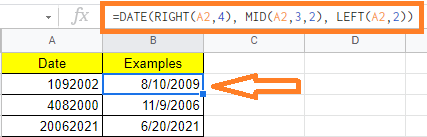## How to Automatically Send Emails from Google Sheets (Using Appscript)

Have you ever thought of sending emails from Google Sheets automatically? If yes, then this article is for you. You can send emails from Google Sheets in two ways. You have the option of using a Google-provided library or a third-party email service provider such as MailChimp or SendGrid.

## How to Automatically Send a Single Email in Google Sheets Using Appscript?

Let us understand how to send an email to a single recipient in Google Sheets. For that, we should create a sample dataset as shown in the image below: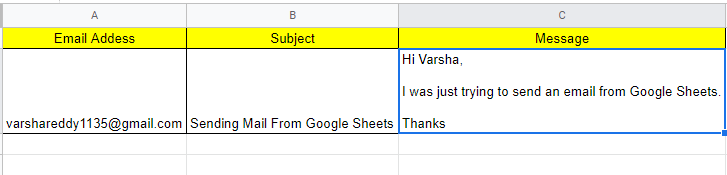### Syntax to Send Email Using AppScript in Google Sheets

The syntax to send an email using Appscript in Google Sheets are explained below:

In the above syntax, the references to the corresponding cells can be used to replace emailAddress, subject, and message.

### Steps to Send Email Using Appscript in Google Sheets

Follow the steps as listed below to send an email from Google Sheets to the respective email using Appscript:

• Step 1: Open the Google Sheets and enter the above sample dataset.
• Step 2: Now click the “Tools” tab in the menubar.
• Step 3: Select “Script Editor” from the drop-down menu.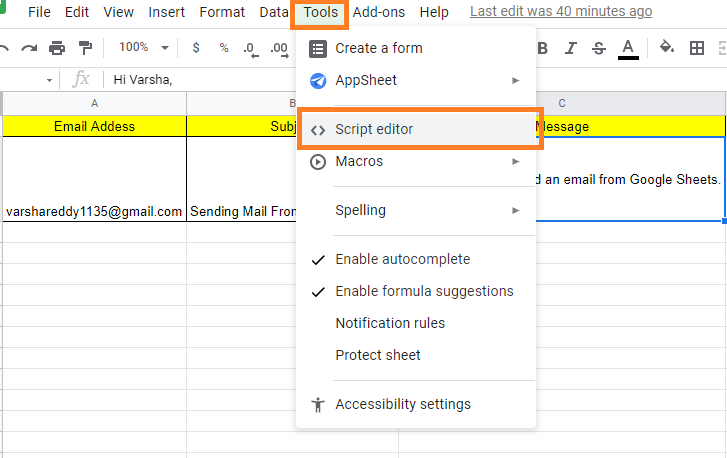• Step 4: Now a script editor screen will open on the screen. Now enter the following code inside the curly braces as shown in the image below the code.

• Step 5: Now click on the “File” and hit the “Save” button.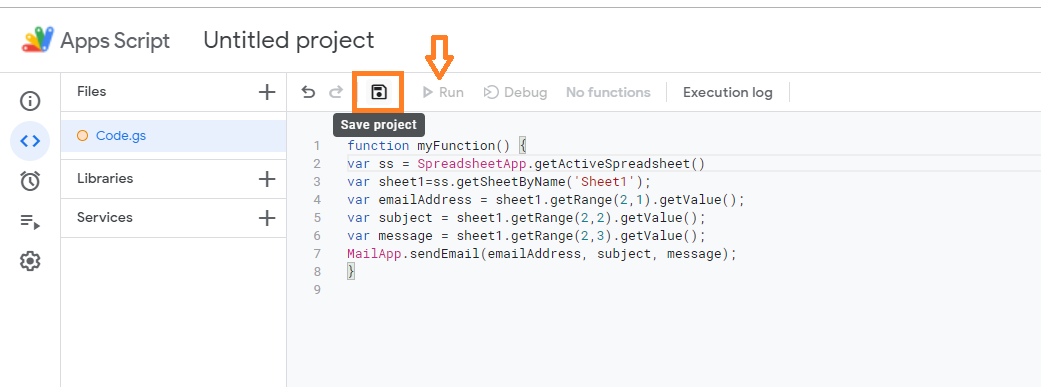• Step 6: Click Run after selecting the function sendEmails from the function dropdown box.
• a. Select Review Permissions from the drop-down menu.
•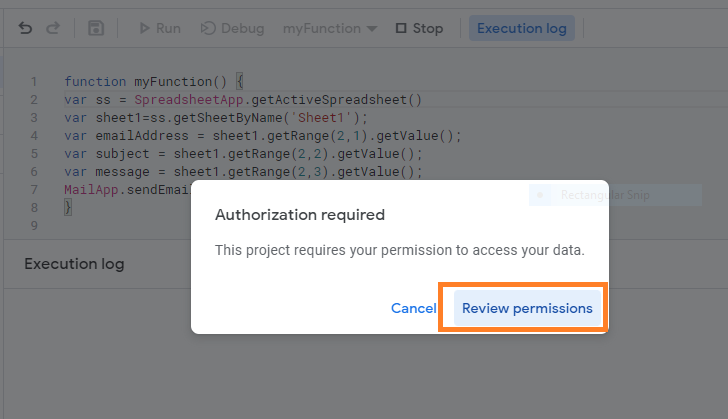• b. You will then be informed that Google has not recognized your App and that you should proceed only if you are familiar with the creator. You are, after all, the author, therefore you clearly don’t mind. Scroll down and click ‘Go to sendEmail‘ after clicking Advanced.
• c. Simply click Allow when you receive the confirmation.
•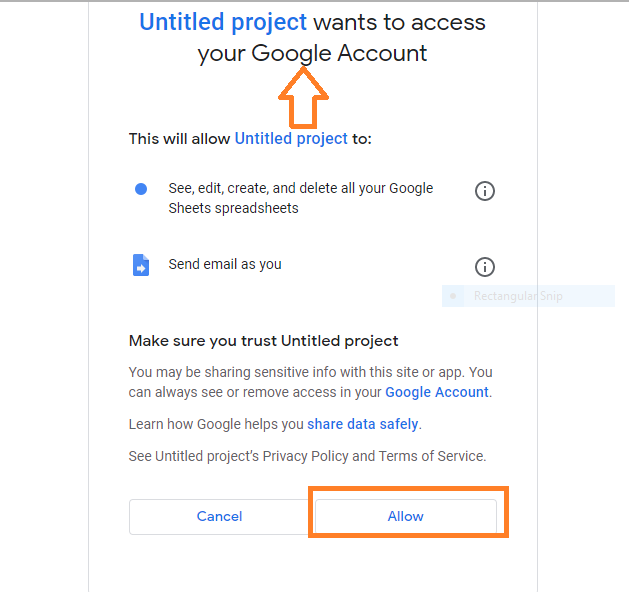Once the Code Running is done, the mail will be sent.

Code Explanation:

We acquire access to the spreadsheet named ‘Sheet1′ in the first two lines and store a handle to it in a variable called sheet1. Three variables – emailAddress, subject, and message – are created in lines 3, 4, and 5. We assign the value from the second row, the first column (cell A2) to the variable emailAddress using Sheet1’s getRange() function. Similarly, the variable subject is assigned to the value in the second row, the second column (B2), and the variable message is assigned to the value in the second row, the third column (C2).

### How to Send an Email to Multiple Mails in Google Sheets?

Follow the steps listed below to send an email to multiple emails addresses:

• Step 1: Create a new empty Spreadsheet.
• Step 2: Add a few data rows. In column A, each row should have an email address and in column B, the email message to be sent to that person. You might want to use your personal email address in column A for testing purposes. Here’s an illustration: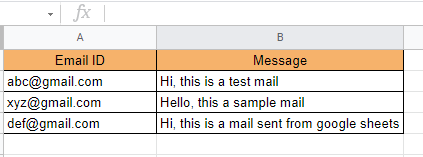• Step 3: Click on the “Tools” tab and select “Script editor” from the drop down menu.
• Step 4: Now Script editor will open towards the right side of the screen. Now copy and paste the following script code.
 /** * Sends emails with data from the current spreadsheet. */ function sendEmails() { var sheet = SpreadsheetApp.getActiveSheet(); var startRow = 2; // First row of data to process var numRows = 2; // Number of rows to process // Fetch the range of cells A2:B3 var dataRange = sheet.getRange(startRow, 1, numRows, 2); // Fetch values for each row in the Range. var data = dataRange.getValues(); for (var i in data) { var row = data[i]; var emailAddress = row; // First column var message = row; // Second column var subject = ‘Sending emails from a Spreadsheet’; MailApp.sendEmail(emailAddress, subject, message); } }

• Step 5: Click on the “Save” button to save the script code.
• Step 6: Choose sendEmails function from the drop-down list of functions, then click Run.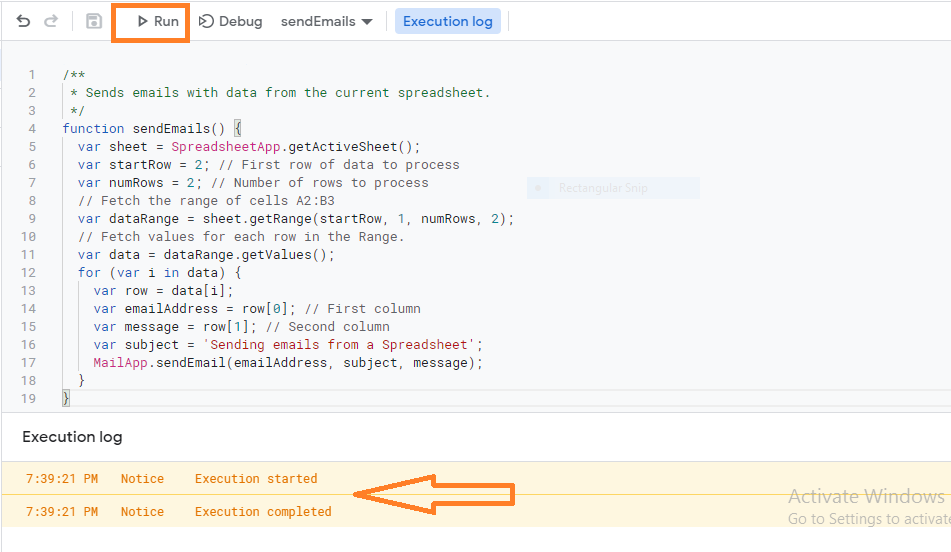• Step 7: Once the code is executed successfully, the email is sent to desired recipients.

### How to Know if Mail Is Sent in Google Sheets?

Every time an email is sent, you might want to mark a cell in each row. It will also allow you to execute the code again later if your script stops working (for example, if there is a flaw in your code, or if you exceed the maximum amount of emails you can send per minute or per day).

For each row, after sendEmail is called, the cells in column C are set to ‘EMAIL SENT.

To execute this, open the script editor from the Tools tab and paste the following code. Soon after pasting the code, click on save and run the code.

 // This constant is written in column C for rows for which an email// has been sent successfully.var EMAIL_SENT = ‘EMAIL_SENT’;/** * Sends non-duplicate emails with data from the current spreadsheet. */function sendEmails2() {  var sheet = SpreadsheetApp.getActiveSheet();  var startRow = 2; // First row of data to process  var numRows = 2; // Number of rows to process  // Fetch the range of cells A2:B3  var dataRange = sheet.getRange(startRow, 1, numRows, 3);  // Fetch values for each row in the Range.  var data = dataRange.getValues();  for (var i = 0; i < data.length; ++i) {    var row = data[i];    var emailAddress = row; // First column    var message = row; // Second column    var emailSent = row; // Third column    if (emailSent !== EMAIL_SENT) { // Prevents sending duplicates      var subject = ‘Sending emails from a Spreadsheet’;      MailApp.sendEmail(emailAddress, subject, message);      sheet.getRange(startRow + i, 3).setValue(EMAIL_SENT);      // Make sure the cell is updated right away in case the script is interrupted      SpreadsheetApp.flush();    }  }}

## How to Create Candlestick Chart in Google Sheets (With Examples)

A chart can depict a set of data values more clearly than a table with rows of numbers. Thus Google Sheets offers various types of charts that we can incorporate with our dataset. One of the most useful charts in Google Sheets is Candlestick Chart. A candlestick chart is a type of visualization tool that may be used to track changes in a variable’s value over time. In this article, let’s understand how to create a candlestick chart with the help of Google Sheet tips provided on this page. Read on to find more.

## What is Candlestick Chart?

A candlestick chart can be used to display the low, high, opening, and closing values of a security over a given time period. With the help of a candlestick chart, we can also get the daily variation in stock prices.

A candlestick chart can also be used to track scientific data, such as rainfall or temperature.

### How to Create Candlestick Chart in Google Sheets?

Consider the following dataset which talks about the monthly stock price.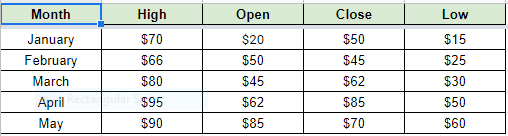Now follow the steps as listed below to create a Candlestick chart in Google Sheets:

• Step 1: Select the dataset columns to create the candlestick chart. In our example, I have selected Month, High, Open, Close, Low.
• Step 2: Click on the “Insert” tab from the menubar.
• Step 3: Now select the “Chart” icon from the drop-down menu.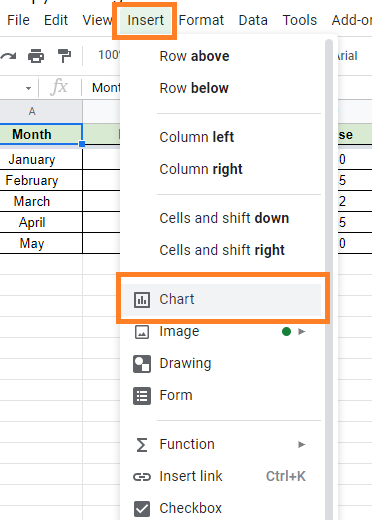• Step 4: The Chart editor will open towards the right side of the screen. Google usually tries to understand the data you have chosen and displays the chart it believes is the best representation of it. It should ideally show a Candlestick chart. If you see a different type of chart, proceed to step 5.
• Step 5: To make a candlestick chart, click on the “Setup” tab and select “Candlestick chart” from the Chart type drop down menu.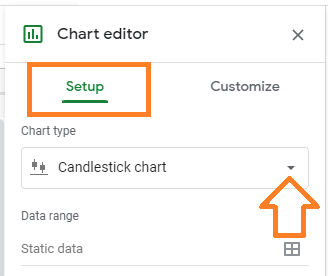• Step 6: Choose the “Candlestick chart” from the chart options that appear. It should be visible in either the “Suggested” or “Other” categories.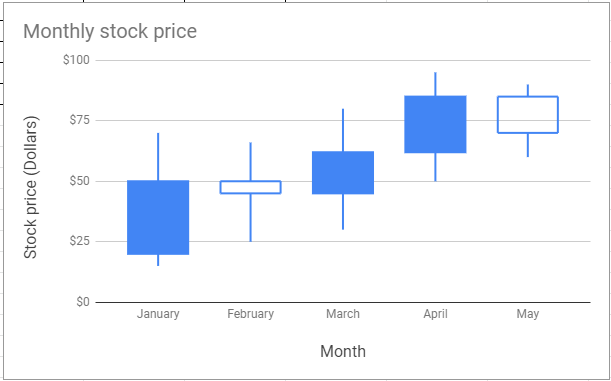### Parameters to Create Candlestick Chart

The list of parameters that generates the candlestick chart in Google Sheets are explained below:

• First Column: Enter a label for the X-axis in the first column.
• Second Column: Enter a number to represent the highest or most extreme value. This value corresponds to the top of the candle’s center line.
• Third Column: In the third column, enter a number as the opening or initial value. This value is one of the candle’s vertical borders.
• Fourth Column: In the fourth column, enter a number to represent the closing or final value. This value is the candle’s second vertical border.
• Fifth Column: Enter a number for the minimum value in the fifth column. This is the value at the bottom of the candle’s center line.
• Rows: A single candlestick marker is represented by each row.

The candlestick chart will be filled if the value of the third column is less than the value of the fourth column. It will be empty otherwise.

### How to Customize Candlestick Chart in Google Sheets?

Although you cannot change the color of the candlesticks, you can make other changes to your chart in Google Sheets. Here’s how to do it:

Generally, the Chart editor has a ‘Customize’ tab where you can enter all of your specifications. Follow the steps listed below to customize your candlestick chart in Google Sheets:

• Step 2: Now double-click on the candlestick chart that needs to be modified.
• Step 3: Here chart editor pane opens towards the right side of the screen. Choose Customize on the right bar.
• Step 4: Here you will be provided with the following set of options. Select one of the following options:
• Chart Style: To change the font and background color.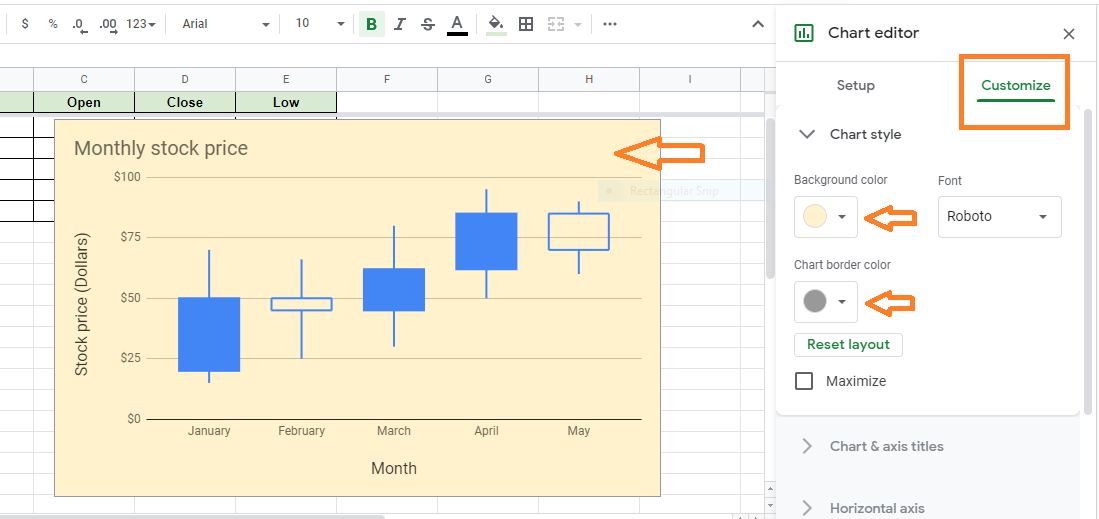• Chart & Axis Title: To edit or format the title text on a candlestick chart.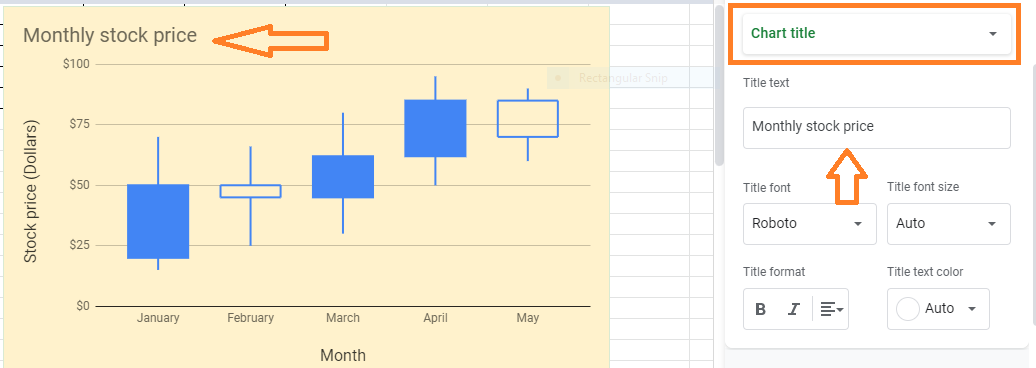• Horizontal Axis: Reverse axis order or edit or format axis text.
• Vertical Axis: Edit or format the axis text, set the minimum and maximum value, or log scale.
• Gridlines: To create and modify gridlines.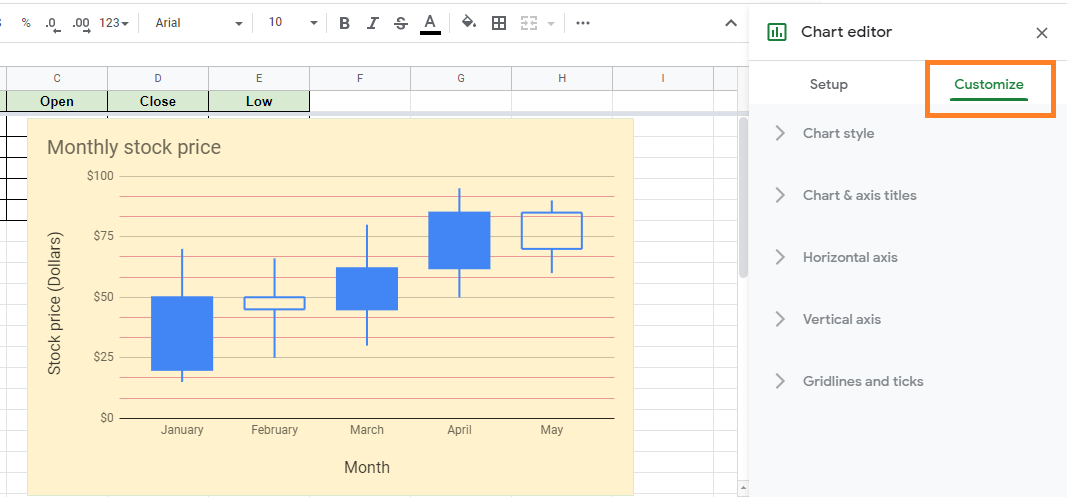## How to Calculate Age in the Google Sheets using the Date of Birth? (with Example)

Have you ever wondered about calculating age using the date of birth in Google Sheets?. If yes, then you are right! With Google Sheets, you can determine the current time, generate charts, and calculate age based on a birthdate. To calculate the age in google sheets, we can use the DATEDIF function. In this article, let’s discuss how to calculate the age using the date of birth with the help of Google Sheets tips provided on this page.

## Calculate the Age in Google Sheets

Google Sheets calculate the age by using the user’s date of birth and the current date.

You can calculate the age in any of the below two methods,

• The total number of years only
• The total number of years, months, and days

Let’s discuss on above in detail.

### Calculate Age in Google Sheets – Years only

To Calculate the Age with years only, you need to specify today’s date and date of birth.

Formula:

=DATEDIF(dob,curr_date,”Y”)

In the above formula,

dob – The Date of birth mentioned with date, month, and year

curr_date – This is the current date i.e., today’s date to calculate the age

“Y” – This represents the Year. The result will be returned in Years only.

Example: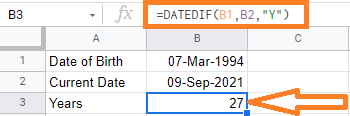In the above example, we are finding the age of the date of birth – 07-Mar-1994.

Cell B1 is having the date of birth and the B2 cell is having the current date. With the expression =DATEDIF(B1, B2, “Y”) we have got 27 years

For getting the Current date, we could also use the TODAY() function in Google Sheets. i.e.,

=DATEDIF(B1,TODAY(), “Y”)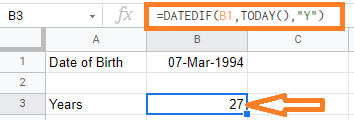In the above image, you could see we are using the TODAY() function to get today’s date.

### Calculate Age in Google Sheets – Years, Months, and Days

To calculate the number of years, months and days, then we need to expand our DATTEDIF Formula.

Let’s first see how to get the individual parts of the result (i.e., the year, month, and day value).

The below formula would give you the year value:

=DATEDIF(dob,curr_date,”Y”)

In the above formula,

dob – The Date of birth mentioned with date, month, and year.

curr_date – This is the current date i.e., today’s date to calculate the age.

“Y” – This represents the Year. The result will be returned in Years only.

Example:In the above example, we are finding the age for the date of birth – 07-Mar-1994.

Cell B1 is having the date of birth and the B2 cell is having the current date. With the expression =DATEDIF(B1, B2, “Y”) we have got 27 years.

The below formula would give you the month value,

=DATEDIF(dob,curr_date,”YM”)

In the above formula,

dob – The Date of birth mentioned with date, month, and year.

curr_date – This is the current date i.e., today’s date to calculate the age.

“YM” – This represents the Month. The result will be returned in Months only.

Example: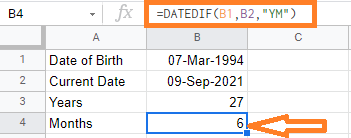In the above example, we are finding the current age month of the date of birth – 07-Mar-1994.

Cell B1 is having the date of birth and the B2 cell is having the current date. With the expression =DATEDIF(B1, B2, “YM”) we have got 6 months.

The below formula would give you the day value,

=DATEDIF(dob,curr_date,”MD”)

In the above formula,

dob – The Date of birth mentioned with date, month, and year

curr_date – This is the current date i.e., today’s date to calculate the age

“MD” – This represents the Day value. The result will be returned in Days only.

Example: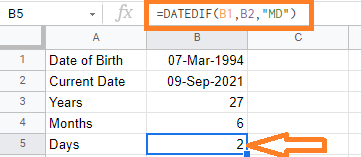In the above example, we are finding the current days difference for the date of birth – 07-Mar-1994.

Cell B1 is having the date of birth and the B2 cell is having the current date. With the expression =DATEDIF(B1, B2, “MD”) we have got 2 days.

The below formula would give you a year, month, and day value

=DATEDIF(B1,B2,“Y”)&” Years “&DATEDIF(B1,B2,“YM”)&” Months & “&DATEDIF(B1,B2,“MD”)&” Days”

In the above formula,

we are concatenating the years, months, and days with the “&” symbol.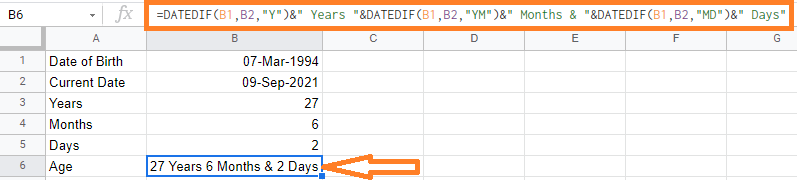You can use the above formula for not only calculating the age but to find the project duration, Employee joining date, and retirement date, and many more.

## How to Convert Formula to Value in Google Sheets?

When working with Google Sheets, we use n number of formulas and functions. However, when we are copying formula applied cells from one cell to another cell, the Google Sheets will show up an error. This error will be shown due to the difference in cell reference numbers that we have used in formulas or functions. Thus in order to avoid this error, we should convert formulas to values in Google Sheets.

## How to Convert Formula to Value in Google Sheets?

In this post, let’s consider the following dataset as an example.

Now let’s understand how to convert formulas to values in Google Sheets with the help of the following two methods:

• Using Paste as Values
• Using Keyboard Shortcut

### Using Paste as Values to Convert Formulas to Values

Consider the following dataset where we need to convert formulas to values: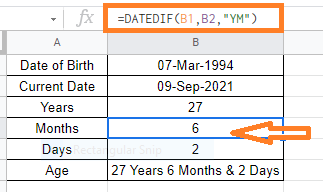Follow the steps listed below to convert formulas to values using the Paste as values method.

• Step 2: Now select the cells that is containing the formulas.
• Step 3: Use “CNTRL + C” to copy the selected cells. Alternatively, right-click and select “Copy“.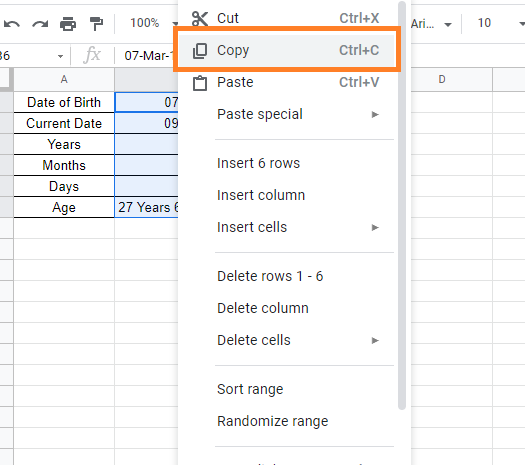• Step 4: Now move the cell, where you like to convert formulas to values.
• Step 5: Right-click and select “Paste Special“.
• Step 6: From the context sub-menu, select “Paste Values Only“.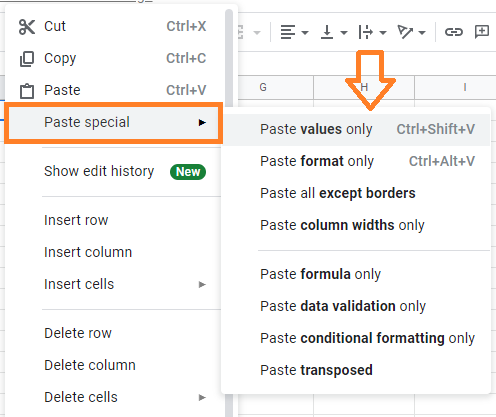In this case, your formulas will be substituted with their returned values, and you will have no formulas at all.

### Using Keyboard Shortcut to Convert Formula to Value

Another easy method to convert formulas to values is using the keyboard shortcut.

Follow the steps listed below to convert formulas to values with the help of Keyboard shortcuts.

• Step 1: Select the cells whose formula needs to be converted to values.
• Step 2: Now copy the cells using “CNTRL+C”. If you are a mac user, press “CMD+C”.
• Step 3: CTRL+SHIFT+V is a keyboard shortcut that you can use (hold down the SHIFT and CTRL keys and then press the V key while the former two keys remain pressed). If you’re using a Mac, instead of CTRL, you can use the CMD key.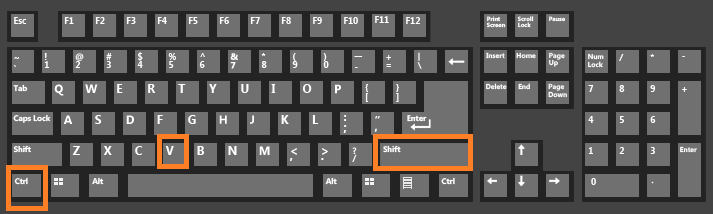### What is the Necessity to Convert Formulas to Values?

Converting a formula to a value can be useful in a variety of situations. The list of reasons why we need to convert formulas to values are explained below:

• When working with large spreadsheets, having too many formulas can cause the processing to slow down. Converting formulas to values can assist speed up spreadsheet processing if you don’t require them any longer.
• When you utilize functions like RAND or RANDBETWEEN, you’ll see that they automatically reload whenever the sheet changes. You might wish to limit yourself to one value. You may just convert the formula to value to prevent the randomly produced cell values from auto-refreshing every time.
• You might need to share a spreadsheet with someone but don’t want to reveal the formulas. It also aids in the conversion of formulas to values in such circumstances.

## How to Convert Time to Military Time Format in Google Sheets?

Google Sheets has several in-built features where we can convert the time into a 12 hour format or military format 24 hours) format. Suppose if you have a dataset where the time is entered in 12 hour format, you can use the built-in function to convert the 12 hour format into 24 format that means military time format.

Let’s understand how to convert time to military time format with the help of Google Sheet tips provided on this page. Read to find more.

## How to Convert to Military Time in Google Sheets?

When you are entering time in Google Sheets, you can affix AM or PM towards the time. By doing so, the Google sheets will consider it as the 12 hour format. However, if you miss out on adding AM or PM next to the time, then Google Sheets by default will consider it as 24 hour format.

Unfortunately, if you have a mix of multiple time formats or need to switch from 12-hour to 24-hour/military time, the default formatting won’t help you.

Now consider the following dataset where we need to convert time to military format.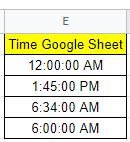Hence follow the steps as listed below to convert time to military time format in Google Sheets.

• Step 1: Select the cells where you would like to convert the time format into the military format.
• Step 2: Click on the “Format” tab in the menubar.
• Step 3: Now select “Number” from the drop-down menu. A sub-menu will open on the screen.
• Step 4: From the submenu select “More Formats” and further select “More date and time formats.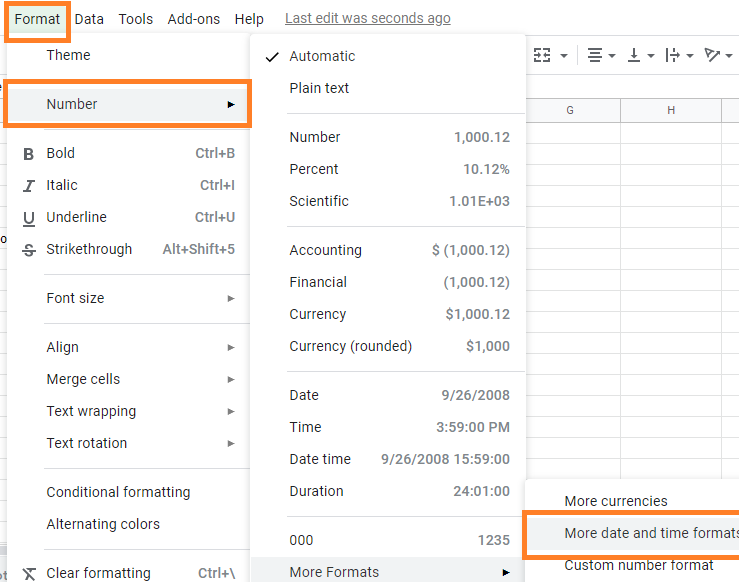• Step 5: Search for the 24-hour formats by scrolling down the pop-up menu. The examples all utilize 1:45:00 PM as an example time so that you can recognize the 24-hour/military time formats as “13:45:00” for the hour/minute/seconds, “13:45” for the hour/minutes, and “8/5 13:45” for the month/day hour/minutes in the military date format. “13:45:00” is used in the example.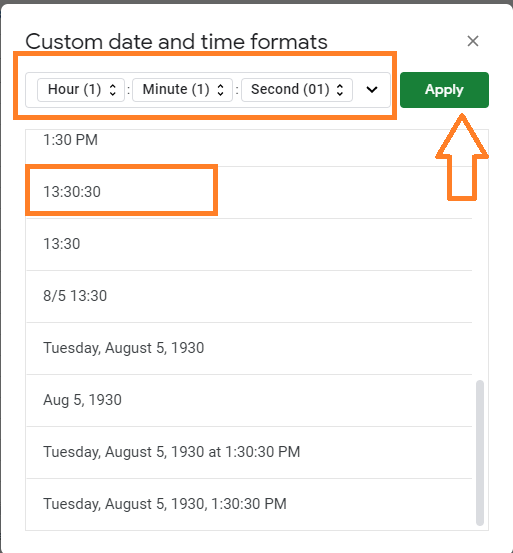• Step 6: Now click on the “Apply” button and you will see the results.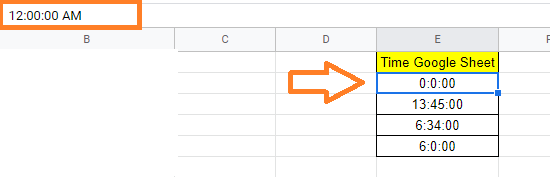### Changing Time Zone and Location in Google Sheets

However, you should be aware that Google Sheets’ default time and date settings are depending on your location.

You can, therefore, change the time zone, location, and even the language of the function. To do so, take the following steps:

• Step 2: From the toolbar, choose “File.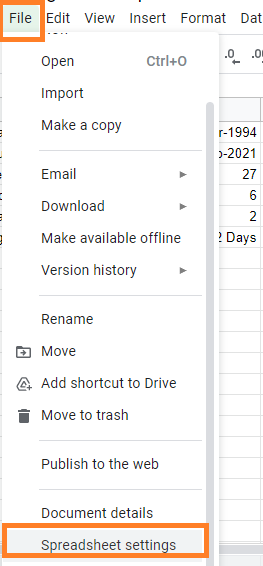• Step 4: Select “General,” then “Locale,” and “Time Zone.”
• Step 5: Now click on “Save settings“.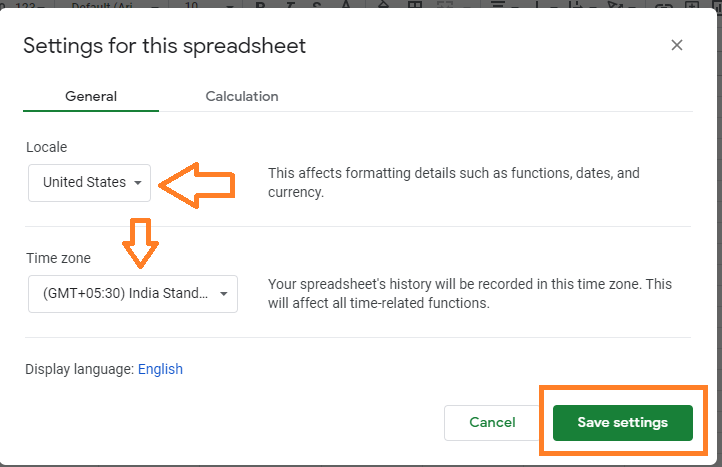### Custom Format and Time Format Settings for Miltary Time

In countries that use the 12-hour clock, the 24-hour clock is sometimes referred to as “military time,” but it is not only used by soldiers.

It is the most prevalent way to tell the time in many countries, in both 12-hour and 24-hour versions.

The most often used 24-hour/military time formats which are available in Google Sheets are as follows. Depending on your needs, you can modify the custom format and time format settings for Miltary time.

 H: MM 13:30 and Hour (1) – 1:00 HH: MM 13:30 and Hour (01) – 01:00 H: MM: SS 13:30:30 and Hour (1) – 1:00:00 HH: MM: SS 13:30:30 and Hour (01) – 01:00:00